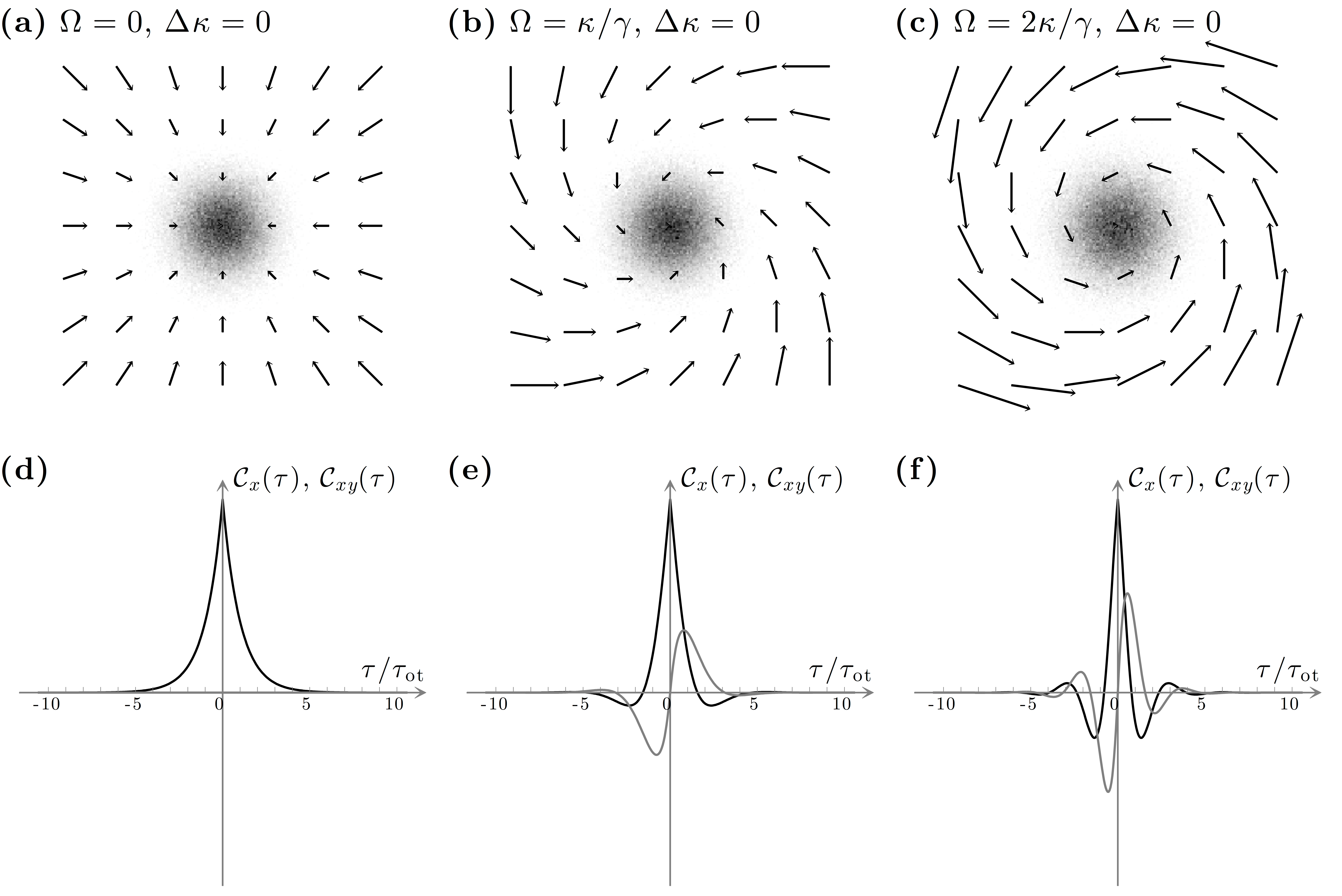# Figure 10.4 — Photonic force microscope in a rotationally symmetric potentialFigure 10.4 — Photonic force microscope in a rotationally symmetric potential. (a)–(c) Photonic torque microscope with a rotationally symmetric harmonic potential, i.e., κx = κy or, equivalently, 􏰀κ = 0, for various values of Ω. The shaded areas show the probability distribution function of the probe particle position in the corresponding force field (arrows). When Ω = 0, the probe movement can be separated along two orthogonal directions. When the (absolute) value of 􏰁 increases, the force field lines bend and the probe movements along the x– and y-directions are not independent any more. (d)-(f ) Corresponding autocorrelation functions Cx(τ) (black solid line) and cross-correlation functions Cxy(τ)(grey solid line) [Eqs. (10.6) and (10.7)]. Note that Cy(τ) = Cx(τ) and Cyx(τ) = Cxy(−τ).